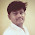## Objective Questions from Transformers:

 The ordinary two winding transformer's primary and secondary windings always have
A.  Different no of turns
B.  Same size of copper wire
C.  A common magnetic circuit
D.  Separate magnetic circuits

 No load test on a transformer is carried out to find
A. Copper loss
B. Magnetising current
C. Magnetising current and no load loss
D. Efficiency of the transformer

 Transformers are rated in KVA instead of KW because
A. Load power factor is often not known
B. KVA is fixed whereas KW depends on load power factor
C. Total transformer loss depends on volt ampere
D. It has become customary

 Transformer cores are laminated in order to
A. Simplify its construction
B. Minimise eddy current loss
C. Reduce cost
D. Reduce hysteresis loss

 A step up transformer increases
A. Voltage
B. Current
C. Power
D. Frequency

 In a two winding transformer, the primary and secondary induced emf E1 & E2 are always
A. Equal in magnitude
B. Anti phase with each other
C. In phase with each other
D. Determined by load on transformer secondary

 In a transformer, the leakage flux of each winding is proportional to the current in that winding because
A. Ohm's law applies to magnetic circuits
B. Leakage paths do no saturate
C. The two windings are electrically isolated
D. Mutual flux is confined to the core

 In a two winding transformer, the emf /turn in secondary winding is always......the induced emf power turn in primary
A. Equal to k times
B. Equal to 1/k times
C. Equal
D. Greater than

 In transformer terminology, the ratio 20:1 indicates that
A. There are 20 turns on primary one turn on secondary
B. Secondary voltage is 1/20th of the primary
C. Primary current is 20 times greater than the secondary current
D. For every 20 turns on primary, there is one turn on secondary

 In performing the short circuit test of a transformer
A. High voltage is usually short circuited
B. Low voltage side is usually short circuited
C. Any side is short circuited with preference
D. None of the above

4:37 PM

#### 2 comments:

1.In transformer terminology, the ratio 20:1 indicates that
A. There are 20 turns on primary one turn on secondary
B. Secondary voltage is 1/20th of the primary
C. Primary current is 20 times greater than the secondary current
D. For every 20 turns on primary, there is one turn on secondary

Ans..D

1.yes D IS CORRECT